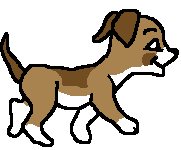# Concept of Dimensions

Below is an example to understand the concept of dimension.

Suppose, your friend lives on the moon and he wants to gift you some books. He asks you your address, but the courier company of the moon does not understand the alphabets they only understand numerical coordinates. So you can give him your position on earth using the concept of dimensions. Dimension is used to define the number of points to point a position of a particular object.

So, you will send three pairs of coordinates to your friend on the moon, 1st is longitude, 2nd is latitude and 3rd is the altitude. These three coordinates are used to define your position on earth. Longitude and latitude are used to define your location and altitudes are used to define your height above the sea level.

The above concept is in reference to digital image processing. So, we are going to deal with this concept of dimension with an image.

## Dimensions of image

Image dimensions have the length and width of a digital image. It does not have depth. An image is measured in pixels. An image is only of 2-dimensional that is why an image is defined as a 2-dimensional signal. Many graphics programmers view and work image in inches or centimeters. Depending on the plane, size of the image can be changed.

## How does television work?

As we have studied above, an image has only 2-dimension. If we want to convert an image to 3-dimension, we will need one more dimension for the conversion. Let time be the 3rd dimension so, 2dimensional images can move on 3rd dimension time.

The same concept is used on television. For example, if we a playing a video, then it is nothing but 2-dimensional pictures moving over the time dimension. As 2-dimensional objects are moving on 3rd dimension object, then we can say it is a three dimensional.

## Different dimensions of signals

#### 1-Dimension Signal

A noisy voice signal is an example of 1 dimension signal. In maths, it can be represented as:

As it is a 1-dimension signal that is why one variable is used.A question arises here that as it is a one-dimensional signal then why it has two axes?

Even, it is a one-dimensional signal but drawn on two-dimensional space. Or we can say that to represent 1-dimensional signal we have used 2-dimensional space.

#### 2-Dimension Signal

Any object which has length and height comes under 2-dimension signal. It has two independent variables.

In maths, it can be represented as:

For example, below picture is a 2-dimensional signal of coordinates X and Y. At each point, an intensity value is given and mapped on the computer screen known as 2-dimensional images.#### 3-Dimension Signal

Any object which has length and height and depth comes under 3-dimension signal. It has three independent variables:

In math, it can be represented as:

Our earth is a 3-dimensional world. A cube is also an example of a 3-dimensional signal#### 4-Dimension Signal

Any object which has length, height, depth and time comes under 4-dimension signal. It has four independent variables.

In math, it can be represented as:

In reality, animated movies are 4D in which 3 dimensions are used and the 4th dimension is time. In animated movies, each character is 3D and moves with respect to the time.For Videos Join Our Youtube Channel: Join Now

### Feedback

• Send your Feedback to feedback@javatpoint.com

## Help Others, Please Share